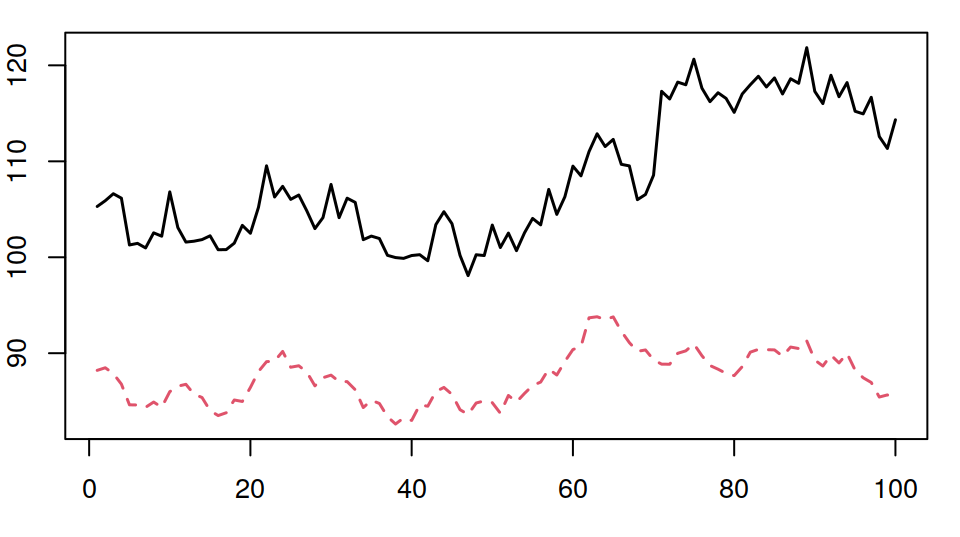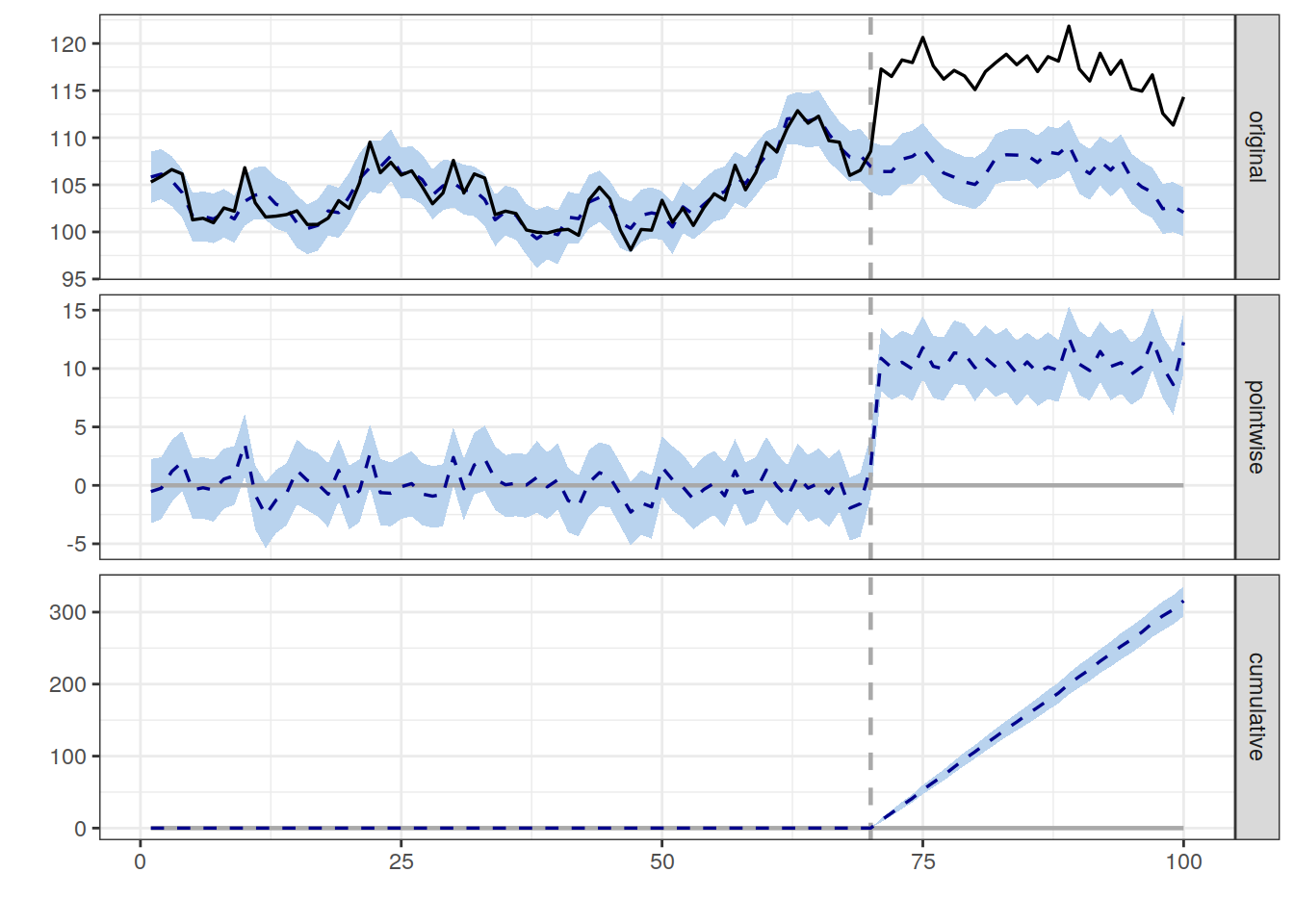### An R package for causal inference using Bayesian structural time-series models

#### What does the package do?

This R package implements an approach to estimating the causal effect of a designed intervention on a time series. For example, how many additional daily clicks were generated by an advertising campaign? Answering a question like this can be difficult when a randomized experiment is not available.

#### How does it work?

Given a response time series (e.g., clicks) and a set of control time series (e.g., clicks in non-affected markets or clicks on other sites), the package constructs a Bayesian structural time-series model. This model is then used to try and predict the counterfactual, i.e., how the response metric would have evolved after the intervention if the intervention had never occurred. For a quick overview, watch the tutorial video. For details, see: Brodersen et al., Annals of Applied Statistics (2015).

#### What assumptions does the model make?

As with all non-experimental approaches to causal inference, valid conclusions require strong assumptions. In the case of CausalImpact, we assume that there is a set control time series that were themselves not affected by the intervention. If they were, we might falsely under- or overestimate the true effect. Or we might falsely conclude that there was an effect even though in reality there wasn't. The model also assumes that the relationship between covariates and treated time series, as established during the pre-period, remains stable throughout the post-period (see `model.args\$dynamic.regression` for a way of relaxing this assumption). Finally, it's important to be aware of the priors that are part of the model (see `model.args\$prior.level.sd` in particular).

#### How is the package structured?

The package is designed to make counterfactual inference as easy as fitting a regression model, but much more powerful, provided the assumptions above are met. The package has a single entry point, the function `CausalImpact()`. Given a response time series and a set of control time series, the function constructs a time-series model, performs posterior inference on the counterfactual, and returns a `CausalImpact` object. The results can be summarized in terms of a table, a verbal description, or a plot.

## 1. Installing the package

`CausalImpact` is available on CRAN and can be installed as follows in an R session:

``install.packages("CausalImpact")``

Once installed, the package can be loaded in a given R session using:

``library(CausalImpact)``

## 2. Creating an example dataset

To illustrate how the package works, we create a simple toy dataset. It consists of a response variable `y` and a predictor `x1`. Note that in practice, we'd strive for including many more predictor variables and let the model choose an appropriate subset. The example data has 100 observations. We create an intervention effect by lifting the response variable by 10 units after timepoint 71.

``````set.seed(1)
x1 <- 100 + arima.sim(model = list(ar = 0.999), n = 100)
y <- 1.2 * x1 + rnorm(100)
y[71:100] <- y[71:100] + 10
data <- cbind(y, x1)``````

We now have a simple matrix with 100 rows and two columns:

``dim(data)``
``##  100   2``
``head(data)``
``````##             y       x1
## [1,] 105.2950 88.21513
## [2,] 105.8943 88.48415
## [3,] 106.6209 87.87684
## [4,] 106.1572 86.77954
## [5,] 101.2812 84.62243
## [6,] 101.4484 84.60650``````

We can visualize the generated data using:

``matplot(data, type = "l")``## 3. Running an analysis

To estimate a causal effect, we begin by specifying which period in the data should be used for training the model (pre-intervention period) and which period for computing a counterfactual prediction (post-intervention period).

``````pre.period <- c(1, 70)
post.period <- c(71, 100)``````

This says that time points 1 ... 70 will be used for training, and time points 71 ... 100 will be used for computing predictions. Alternatively, we could specify the periods in terms of dates or time points; see Section 5 for an example.

To perform inference, we run the analysis using:

``impact <- CausalImpact(data, pre.period, post.period)``

This instructs the package to assemble a structural time-series model, perform posterior inference, and compute estimates of the causal effect. The return value is a `CausalImpact` object.

## 4. Plotting the results

The easiest way of visualizing the results is to use the `plot()` function that is part of the package:

``plot(impact)``By default, the plot contains three panels. The first panel shows the data and a counterfactual prediction for the post-treatment period. The second panel shows the difference between observed data and counterfactual predictions. This is the pointwise causal effect, as estimated by the model. The third panel adds up the pointwise contributions from the second panel, resulting in a plot of the cumulative effect of the intervention.

Remember, once again, that all of the above inferences depend critically on the assumption that the covariates were not themselves affected by the intervention. The model also assumes that the relationship between covariates and treated time series, as established during the pre-period, remains stable throughout the post-period.

## 5. Working with dates and times

It is often more natural to feed a time-series object into `CausalImpact()` rather than a data frame. For example, we might create a `data` variable as follows:

``````time.points <- seq.Date(as.Date("2014-01-01"), by = 1, length.out = 100)
data <- zoo(cbind(y, x1), time.points)
head(data)``````
``````##                   y       x1
## 2014-01-01 105.2950 88.21513
## 2014-01-02 105.8943 88.48415
## 2014-01-03 106.6209 87.87684
## 2014-01-04 106.1572 86.77954
## 2014-01-05 101.2812 84.62243
## 2014-01-06 101.4484 84.60650``````

We can now specify the pre-period and the post-period in terms of time points rather than indices:

``````pre.period <- as.Date(c("2014-01-01", "2014-03-11"))
post.period <- as.Date(c("2014-03-12", "2014-04-10"))``````

As a result, the x-axis of the plot shows time points instead of indices:

``````impact <- CausalImpact(data, pre.period, post.period)
plot(impact)``````